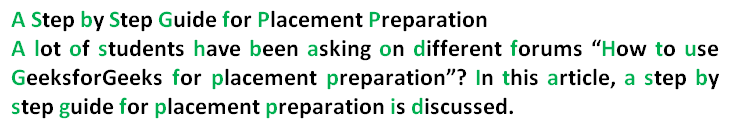# Null Cipher

• Difficulty Level : Easy
• Last Updated : 14 Jul, 2022

A null cipher, also known as concealment cipher, is an ancient form of encryption where the plaintext is mixed with a large amount of non-cipher material. Today it is regarded as a simple form of steganography, which can be used to hide ciphertext.

There are various options of using the Null Cipher. Here we are taking the first letter from each word successively. The pattern can be chosen to increase the cryptography level Other options can be:

• Taking last letters from each word.
• Taking the letter from the particular position
• Using the pattern (1, 2, 3, 1, 2, 3 [each letter in each word]). You can use some other pattern also.
• Positing of the significant letters next to or at certain intervals from punctuation marks or particular characters.

Null Cipher taking the first letter from each word successivelyMore Examples of messages containing null ciphers:

```Input will be one paragraph or sentence without any newline.

Input : News Eight Weather: Tonight increasing snow.
Unexpected precipitation smothers eastern
towns. Be extremely cautious and use snowtires
especially heading east. The [highway is not]
knowingly slippery. Highway evacuation is
suspected. Police report emergency situations
in downtown ending near Tuesday.

Explanation:
Taking the first letter in each word successively yields
the real message.
Here we are converting decoded message to lowercase.

News Eight Weather: Tonight Increasing Snow.
Unexpected Precipitation Smothers Eastern
Towns. Be Extremely Cautious And Use Snowtires
Especially Heading East. The [Highway Is Not]
Knowingly Slippery. Highway Evacuation Is
Suspected. Police Report Emergency Situations
In Downtown Ending Near Tuesday.

Output :
After Deciphered : newtisupsetbecausehethinksheispresident

After breaking the words manually the output will be:
Newt is upset because he thinks he is President```

Implementation:

## C++

 `// CPP program to decode NULL CIPHER``#include ``using` `namespace` `std;``string decode(string str)``{``    ``// Store the decoded string.``    ``string res = ``""``;``    ``// found variable is used to tell that the encoded``    ``// encoded character is found in that particular word.``    ``bool` `found = ``false``;` `    ``for` `(``int` `i = 0; i < str.size(); i++)``    ``{``        ``// Set found variable to false whenever we find``        ``// whitespace, meaning that encoded character for``        ``// new word is not found``        ``if` `(str[i] == ``' '``)``        ``{``            ``found = ``false``;``            ``continue``;``        ``}``        ``if` `(!found)``        ``{``            ``if` `(str[i] >= ``'A'` `&& str[i] <= ``'Z'``)``            ``{``                ``res += str[i] + 32;``                ``found = ``true``;``            ``}``            ``else` `if` `(str[i] >= ``'a'` `&& str[i] <= ``'z'``)``            ``{``                ``res += str[i];``                ``found = ``true``;``            ``}``        ``}``    ``}``    ``return` `res;``}``// Driver code``int` `main()``{``    ``string in;``    ``in = ``"A Step by Step Guide for Placement Preparation by GeeksforGeeks"``;` `    ``cout << ``"Enciphered Message: "``;` `    ``// Function call``    ``cout << decode(in) << endl;``    ``return` `0;``}`

## Java

 `// Java program to decode NULL CIPHER``class` `GFG``{` `    ``// Function to decode the message.``    ``static` `String decode(String str)``    ``{``        ``// Store the decoded string``        ``String res = ``""``;` `        ``// found variable is used``        ``// to tell that the encoded``        ``// encoded character is``        ``// found in that particular word.``        ``boolean` `found = ``false``;``        ``for` `(``int` `i = ``0``; i < str.length(); i++)``        ``{``            ``// Set found variable to false``            ``// whenever we find``            ``// whitespace, meaning that``            ``// encoded character for``            ``// new word is not found``            ``if` `(str.charAt(i) == ``' '``)``            ``{``                ``found = ``false``;``                ``continue``;``            ``}``            ``if` `(!found)``            ``{``                ``if` `((str.charAt(i) >= ``'A'` `&& str.charAt(i) <= ``'Z'``) ||``                    ``(str.charAt(i) >= ``'a'` `&& str.charAt(i) <= ``'z'``))``                ``{``                    ``res += Character.toString(str.charAt(i));``                    ``found = ``true``;``                ``}``            ``}``        ``}``        ``return` `res.toLowerCase();``    ``}` `    ``// Driver Code``    ``public` `static` `void` `main(String[] args)``    ``{``        ``String in;``        ``in = ``"A Step by Step Guide for Placement Preparation by GeeksforGeeks"``;``        ``System.out.println(``"Enciphered Message: "` `+ decode(in));``    ``}``}` `// This code is contributed by Vivek Kumar Singh`

## Python3

 `# Python program to decode NULL CIPHER` `# Function to decode the message.``def` `decode(string):``    ` `    ``# Store the decoded string``    ``res ``=` `""` `    ``# found variable is used``    ``# to tell that the encoded``    ``# encoded character is``    ``# found in that particular word.``    ``found ``=` `False` `    ``for` `character ``in` `string:` `        ``# Set found variable to false``        ``# whenever we find``        ``# whitespace, meaning that``        ``# encoded character for``        ``# new word is not found``        ``if` `character ``=``=` `' '``:``            ``found ``=` `False``            ``continue` `        ``if` `not` `found:``            ``if` `character>``=``'A'` `and` `character<``=``'Z'` `or` `character>``=``'a'` `and` `character<``=``'z'``:``                ``res ``+``=` `character``                ``found ``=` `True` `    ``return` `res.lower()` `# Driver code``if` `__name__ ``=``=` `"__main__"``:``    ``input` `=` `"A Step by Step Guide for Placement Preparation by GeeksforGeeks"``    ``print``(``"Enciphered Message:"``,decode(``input``))` `# This code is contributed by Vivek Kumar Singh`

## C#

 `// A C# program to decode NULL CIPHER``using` `System;``    ` `class` `GFG``{` `    ``// Function to decode the message.``    ``static` `String decode(String str)``    ``{``        ``// Store the decoded string``        ``String res = ``""``;` `        ``// found variable is used``        ``// to tell that the encoded``        ``// encoded character is``        ``// found in that particular word.``        ``Boolean found = ``false``;``        ``for` `(``int` `i = 0; i < str.Length; i++)``    ``{``        ``// Set found variable to false whenever we find``        ``// whitespace, meaning that encoded character for``        ``// new word is not found``        ``if` `(str[i] == ``' '``)``        ``{``            ``found = ``false``;``            ``continue``;``        ``}``        ``if` `(!found)``        ``{``            ``if` `(str[i] >= ``'A'` `&& str[i] <= ``'Z'``)``            ``{``                ``res += (``char``)(str[i] + 32);``                ``found = ``true``;``            ``}``            ``else` `if` `(str[i] >= ``'a'` `&& str[i] <= ``'z'``)``            ``{``                ``res += (``char``)str[i];``                ``found = ``true``;``            ``}``        ``}``    ``}``    ``return` `res;``    ``}` `    ``// Driver Code``    ``public` `static` `void` `Main(String[] args)``    ``{``        ``String str;``        ``str = ``"A Step by Step Guide for Placement Preparation by GeeksforGeeks"``;``        ``Console.WriteLine(``"Enciphered Message: "` `+ decode(str));``    ``}``}` `// This code has been contributed by 29AjayKumar`

## Javascript

 ``

Output

`Enciphered Message: asbsgfppbg`

This article is contributed by Sachin Bisht. If you like GeeksforGeeks and would like to contribute, you can also write an article using write.geeksforgeeks.org or mail your article to review-team@geeksforgeeks.org. See your article appearing on the GeeksforGeeks main page and help other Geeks.

My Personal Notes arrow_drop_up